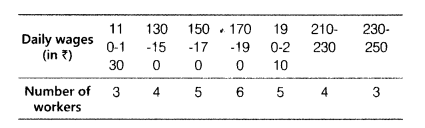# Below is the frequency distribution of daily wages (in ₹) of 30 workers in a certain factory

Given below is the frequency distribution of daily wages (in ₹) of 30 workers in a certain factoryA worker is selected at random. Find the probability that his wage is
(i) less than ₹ 150.
(ii) atleast ₹ 210.
(iii) more than or equal to ₹ 150 but less than
₹ 210.

Total number of workers = 30 [given]
(i) Number of workers Whose wage is less than ₹ 150
=3+4=7
∴ Probability that a worker gets wage less than ₹150
=7/30
(ii) Number of workers whose wage is atleast ₹ 210
=4+3=7
Probability that a worker gets wage of atleast ₹ 210
=7/30
(iii) Number of workers whose wage is more than or equal to₹ 150 but less than ₹ 210
= 5+6+5 = 16
∴ Probability that a worker gets wage is more than or equal to ₹ 150 but less than ₹ 210 =16/30=8/5